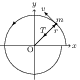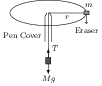# Angular Momentum of a Particle

## Problems from IIT JEE

Problem (IIT JEE 1993): A stone of mass $m$, tied to the end of a string, is whirled around in a horizontal circle. (Neglect the force due to gravity). The length of the string is reduced gradually keeping the angular momentum of the stone about the centre of the circle constant. Then, the tension in the string is given by $T=Ar^n$, where $A$ is a constant, $r$ is the instantaneous radius of the circle. Find $n$.

Solution:Consider motion of the particle of mass $m$ at some time instant $t$. The position vector $\vec{r}$ and the velocity vector $\vec{v}$ of the particle are perpendicular to each other (see figure). The angular momentum of a particle about the centre O is given by $\vec{L}=m\,\vec{r}\times\vec{v}=mvr\,\hat{k}$. The tension in the string $T$ provides centripetal acceleration to the particle i.e., \begin{align} T=\frac{mv^2}{r}=\frac{m}{r}\left(\frac{L}{mr}\right)^2=\left(\frac{L^2}{m}\right)r^{-3}.\nonumber \end{align}Comparing with $T=Ar^{n}$, we get $n=-3$. The readers are encouraged to make a small demo to feel the physics. Take a ball pen and remove the refill so that you can pass a thread through the pen cover. Take a thread and tie one of its end to an eraser, pass the other end through the pen cover, and tie a larger mass (key ring etc) to the other end (see figure). Hold the pen cover and rotate the eraser in horizontal circle. Increase the rotation speed till mass $M$ just starts lifting up. At this instant $T=Mg$. Increase the speed further till mass $M$ touches the pen. Now, pull the mass $M$ downward and see what happens to the speed of mass $m$. Try various ways to feel the relation between $T$, $r$, and $v$.